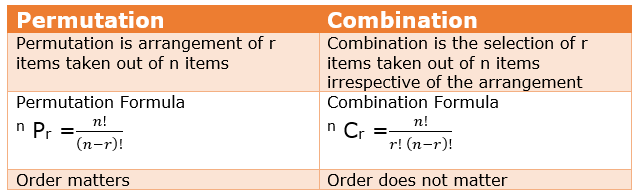# Combination Formula

## Introduction to Combination:

• We have studied about arrangement i.e permutation in the previous chapter
• We learnt that arrangement of r objects taken from n objects is given by
$^{n}P_{r}=\frac {n!}{(n-r)!}$
We would be discussing the combination in this chapter
• Combination is defined  as the different selection made by taking some objects out of  many objects irrespective of their arrangement
So arrangement does not matter in Combination while it matters in Permutation
Example
We have three letter XYZ,
We have to  select two letters and arrange it
Different arrangement would be
XY YZ ZX YX,ZY,XZ
So this is permuatation
Now if we have to just select two letter and do not worry about the arrangement then
XY, YZ ,ZX
This is combination
• Combination  generally happens with committee selection  problems as it does not matter how your committee is arranged. In other words, it generally does not matter whether you think about your committee as Vijay, Arun and Nita or as Nita, Vijay and Arun. In either case, the same three people are in the committee. Contrast this with permutations examples in previous where arrangement matters

## Combination Formula

A formula for the number of possible combinations of $r$ objects from a set of $n$ objects. This is written in any of the ways shown below
$^{n}C_{r} =\frac {n!}{r! (n-r)!}$
Proof:
Let $x$ be the combination of $n$ distinct objects taken $r$ at a time.
Now lets us consider one of the $x$ ways. There are $r$ objects in this one way which can be arranged in $r!$ways . Similarly all these $x$ ways can be arranged in this manner
So permutation of the n things taken r at a time will be given as
$^{n}P_{r}=x r!$
or $x=\frac { n!}{r! (n-r)!}$
$^{n}C_{r} =\frac {n!}{r! (n-r)!}$
Example:
Eleven students put their names on slips of paper inside a box. Three names are going to be taken out. How many different ways can the three names be chosen?
Solution
As the arrangement does not matter, so different ways are
$^{n}C_{r} =\frac {n!}{r! (n-r)!}$
$^{11}C_{3} =\frac {11!}{3! (11-3)!}=165$

## Difference Between Permutation and Combination## Properties of Combination Formula

$^{n}C_{r} =\frac {n!}{r! (n-r)!}$
 1 $^{n}C_{n} = 1$ , $^{n}C_{0} = 1$ as $^{n}C_{n} =\frac {n!}{n! (n-n)!}= \frac {1}{0!} =1$ 2 for $0 \leq r <\leq n$ , we have $^{n}C_{r} = ^{n}C_{n-r}$ As $^{n}C_{r} =\frac {n!}{r! (n-r)!}$ $^{n}C_{n-r}= \frac {n!}{(n-r)! (n-n+r)!}= \frac {n!}{r! (n-r)!}$ 3 $^{n}C_{x} = ^{n}C_{y}$ Then $x=y$ or $x+y=n$ 4 $^{n}C_{r}+ ^{n}C_{r-1}= ^{n+1}C_{r}$ This property is called Pascal law’s 5 for $1 \leq r <\leq n$ , we have $^{n}C_{r} =\frac {n}{r} ^{n-1}C_{r-1}$ 6 for $1 \leq r <\leq n$, we have $n ^{n-1}C_{r-1} =(n-r+1) ^{n}C_{r-1}$

## Solved Examples

Question -1
$^{2n}C_{3} : ^{n}C_{3} =11 :1$
Find the value of n
Solution:
Writing this in factorial form
$\frac {\frac {2n!}{3! (2n-3)!}}{\frac {n!}{3!(n-3)!}} =\frac {11}{1}$
Simplifying it we get
$\frac {2n(2n-1)(2n-2)}{n(n-1)(n-2)}=\frac {11}{1}$
Solving this
$n=6$
Question-2
Over the weekend, your family is going on vacation, and your mom is letting you bring your favorite video game console as well as five of your games. How many ways can you choose the five games if you have 10 games in all?
Solution
Applying combination formula
$^{n}C_{r} =\frac {n!}{r! (n-r)!}$
$^{10}C_{5}=\frac {10!}{5! (10-5)!}=\frac {10 \times 9 \times 8 \times 6}{5 \times 4 \times 3 \times 2 \times 1}=36$
Question-3
In how many ways can a T20 eleven can be chosen out of batch of 15 players if
1. There is no restriction on the selection
2. A particular player is always chosen
3. A particular player is never chosen
Solution:
1. If 11players to be chosen from 15 ,then $^{15}C_{11}=1365$
2.  If the particular player is always choosen,then we need to choose 10 players from remaining 14 players $^{14}C_{10} =1001$
3. If the particular player is never choosen,then we need to choose 11 players from remaining 14 players $^{14}C_{11} =364$

## Related Topics

Go back to Class 12 Main Page using below links